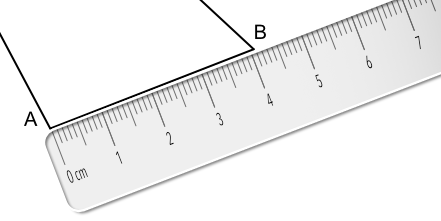Measuring Lines

# Measuring Lines

Basic,

Lines on diagrams are measured using a ruler or scale. When measuring a line on a diagram, ensure that the zero mark on the ruler is at one end of the line, and read off the measurement at the other end of the line. Make sure that the ruler lies parallel to, and close to, the line.

Check the numbers on the scale. Most rulers measure in centimetres: the numbers on the scale indicate the number of centimetres. The small marks are tenths of a centimetre, or millimetres. In the example below, the measurement is 8.3 centimetres.

The Imperial measurement used for drawing is inches. Measurements smaller than an inch will be shown as piths of an inch, tenths of an inch or sixteenths of an inch.

## Examples

1. What is the length of the line AB? Give your answer in centimetres.Place the ruler. Read along to the 4 for 4 centimetres. There is one small unit, which is a tenth of a centimetre: 4 + 0.1 = 4.1cm.

2. The height of this rectangle is 3.1cm. What is the length of the rectangle?